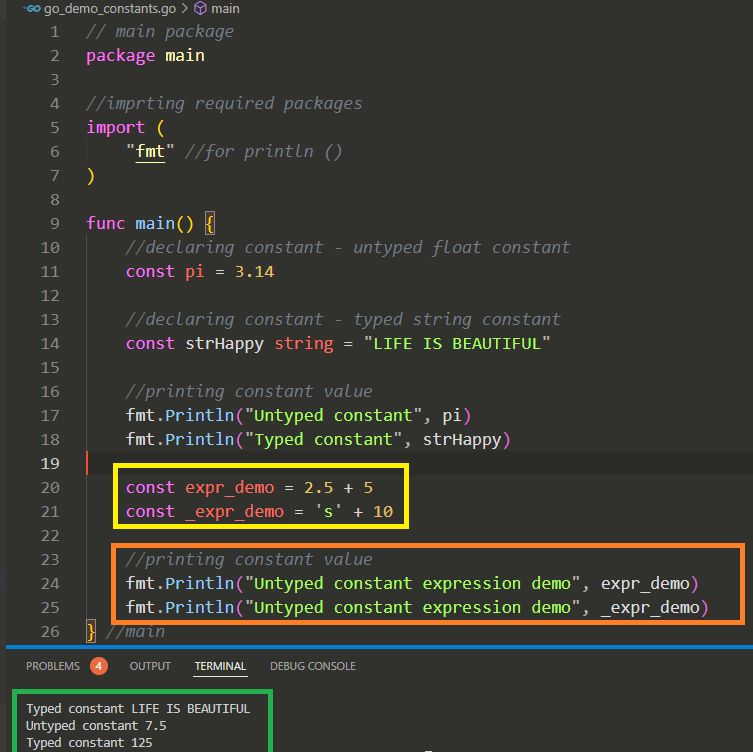# The Go Language – Exploring Constants – part 5Exploring Constants in Go - demonstration of Untyped constants in expressions

Hi All,

We are continuing Go language series. In following previous articles we explored introduction to Go, wrote our first Go program, went through basic data types in Go and started with exploring strings in Go

• What are Constants in Go
• Types of Constants in Go
• Using Constants in expressions
• Constant generator – iota

What are constants in Go

• Constants are used for fixed values. For example value of “pi” in maths – it always remain same, never change
• Constants are used when we need to use it through out project and doesn’t want to change the value – also avoid accidental change in value
• Declaring constant – constants can be declared in Go by using “const” keyword as

``const <constant_name> [data type] = "fixed value"``

• Examples
• const pi = 3.14 //approximate
• const strHappy string = “LIFE IS BEAUTIFUL”
• Data Type is optional – if we didn’t specified data type then its untyped constant – and based on right hand side value type is considered

• Once we declared constants we can change there values , if we try to change the constant value we get error / panic as

can not assigned to pi

• We can not use “:=” to declare Constants

Types of Constants in Go

• Typed constants
• Constants which are declared with specific data type
• Untyped constants
• If we didn’t declare constant with specific data type those are untyped constants
• There are untyped boolean, untyped integer, untyped rune, untyped float, untyped complex and untyped string – untyped constants
• Untyped constants allows us to use them in expression like

``````        //declaring constant - untyped float constant
const pi = 3.14

//declaring constant - typed string constant
const strHappy string = "LIFE IS BEAUTIFUL"``````

• In Go, we are not allowed to use mixed type of variables in expression like

``````var intNum int = 200 //variable of type int
var int64Num = 500 // variable of type int64

var result = intNum + int64Num // compile time error``````

• To work above code we need to do explicitly type casting as

``````var intNum int = 200 //variable of type int
var int64Num = 500 // variable of type int64

var result = intNum + int(int64Num)``````

• But we can use mixed type in expressions by using untyped constants

``````//Untyped constants used in expression
const expr_demo = 2.5 + 5

const _expr_demo = 's' + 10``````

• If we compare two different untyped constants – result will be always untyped boolean constant as

``const boolResult = 5 > 7.5  // false - untyped boolean constant``

• If we use same typed constants in expression – result will be of same type as

``const boolSameTypeResult = 10/3 //result is-3-untyped integer constant``

• If in expression, if operands are of different types then result will be operands type that is bigger as – int < rune < float < complex

``const boolSameTypeResult = 10.0/3 //result is-3.3-float constant``
• Constant generator – iota
• iota is used to generate sequence of related values starting with Zero (0) and increments value by 1 for each item
• Example
• in time package, there is constant of type weekday represent the days of week, starting with Sunday with value Zero (0) as below in code
• Here value for Sunday will be 0 , Monday – 1…

``````	type Weekday int

const (
Sunday Weekday = iota
Monday
Tuesday
Wednesday
Thursday
Friday
Saturday
)``````

Thanks for reading !!! Any discussion / suggestions / thoughts / questions are welcome!!!

HAVE A GREAT TIME AHEAD !!! LIFE IS BEAUTIFUL 🙂